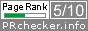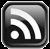# LEARN MS-ACCESS TIPS AND TRICKS

Learn Microsoft Access Advanced Programming Techniques, Tips and Tricks.

### ROUNDDOWN Function of Excel

We have introduced the ROUNDUP() Function of Excel in Microsoft Access earlier.  It works like the ROUND() Function except that the number is always rounded up.  When a number of digits are greater than 0 (zero), then the number is rounded up to the specified number of decimal places. If a number of digits specified are 0 (zero), then the number is rounded up to the nearest integer.

Visit the following link for more details on Roundup() Function:

The ROUNDDOWN() Function of Excel does the opposite of the ROUNDUP() Function.  When the number of digits specified is greater than 0 (zero) then the number is rounded down to the specified number of Decimal Places.  If the number of digits specified is 0 (zero) then the number is rounded down to the nearest integer.

Syntax:

ROUNDDOWN(Number, num_digits)

Number: RequiredAny Real Number.

num_digits: Required, Number of Digits the Number to Round Down to.

```Public Function ROUNDDOWN(ByVal Num As Double, ByVal num_digits As Integer) As Double
'-------------------------------------------------
'ROUNDDOWN() Function of Excel Redefined in MS-Access
'Author: apr pillai
'Date  : Sept 2019
'-------------------------------------------------

Dim S1 As Integer, S2 As Integer

On Error GoTo ROUNDDOWN_Err
S1 = Sgn(Num)
S2 = Sgn(num_digits)

Select Case S1
Case 0
ROUNDDOWN = 0
Exit Function
Case 1
Select Case S2
Case 0
ROUNDDOWN = Int(Num) * S1
Case 1
ROUNDDOWN = (Int(Num * (10 ^ num_digits)) / 10 ^ num_digits) * S1
Case -1
num_digits = Abs(num_digits)
ROUNDDOWN = Int(Num / (10 ^ num_digits)) * 10 ^ (num_digits) * S1
End Select
Case -1
Select Case S2
Case 0
ROUNDDOWN = Int(Abs(Num)) * S1
Case 1
ROUNDDOWN = (Int(Abs(Num) * (10 ^ num_digits)) / 10 ^ num_digits) * S1
Case -1
num_digits = Abs(num_digits)
ROUNDDOWN = (Int(Abs(Num) / (10 ^ num_digits)) * 10 ^ num_digits) * S2
End Select
End Select

ROUNDDOWN_Exit:
Exit Function

ROUNDDOWN_Err:
MsgBox Err & " : " & Err.Description, , "ROUNDDOWN()"
Resume ROUNDDOWN_Exit
End Function

```

The ROUNDDOWN() Function is not field-tested for accuracy, use it at your own risk.

The Function is developed based on the sample output given in the Microsoft Help Document. The Microsoft Excel Help Document extract is reproduced below for your information.

=ROUNDDOWN(3.2, 0)Rounds 3.2 down to zero decimal places.3
=ROUNDDOWN(76.9,0)Rounds 76.9 down to zero decimal places.76
=ROUNDDOWN(3.14159, 3)Rounds 3.14159 down to three decimal places.3.141
=ROUNDDOWN(-3.14159, 1)Rounds -3.14159 down to one decimal place.3.1
=ROUNDDOWN(31415.92654, -2)Rounds 31415.92654 down to 2 decimal places to the left of the decimal point.31400
Share:

Comments subject to moderation before publishing.

## TranslateSubscribe in a readerYour email address:

Delivered by FeedBurner